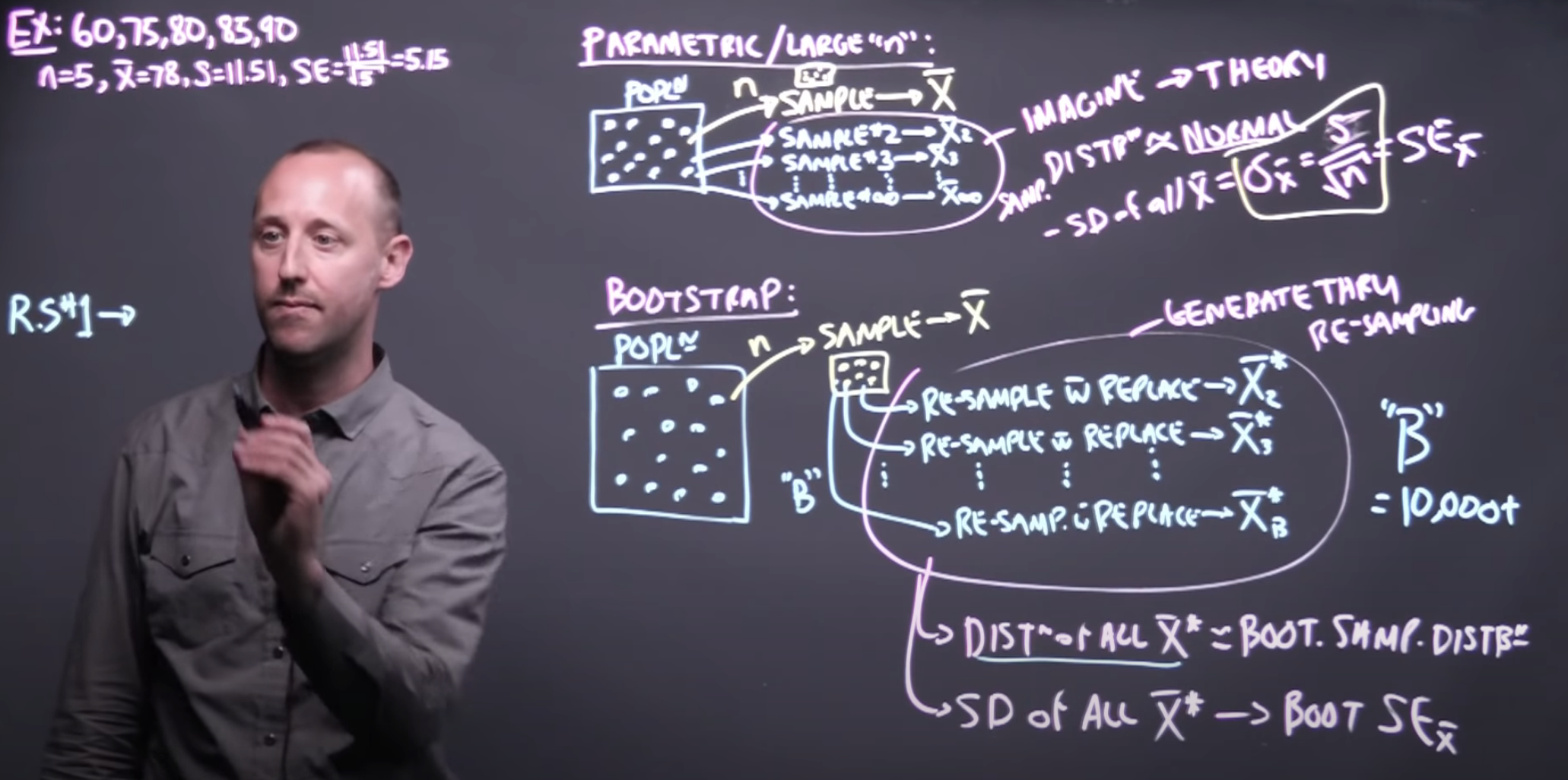# Bootstraping as a technique for building confidence intervals

20210114223129

When you want to extract a value from a series of data (like a mean, a prediction from multiple weak classifiers - RandomForests - etc.. ) you may also need to know a confidence interval for that number.

One analytical way of doing this is by employing a technique called Bootstrapping. This is a good whiteboard explanation for it.The procedure is roughly the following:

• do multiple times (at least 10 000) the following:
• resample with replacement a number of $n$ elements from the original dataset (presumed to be of size $n$ as well)
• on the resulted series compute your desired metric
• at the end of the above loop you should have a series of metrics computed on a list of synthetic (resampled) data akin to multiple Monte-Carlo simulations.
• On the above series of metrics, compute the histogram as it should resemble a normal distribution (bell shaped) - by the Law Of Large Numbers.
• Compute the parameters of this normal distribution, the mean and the std to see
• where your confidence interval starts and end ( [-2std, +2std] encompass 96.7% observations, [-3std, 3std] encompass 99.7% observations, etc..)

Find also here a refresher on confidence intervals computed directly on the std of a normal class

This video from Khan academy is also great at explaining p-value calculation